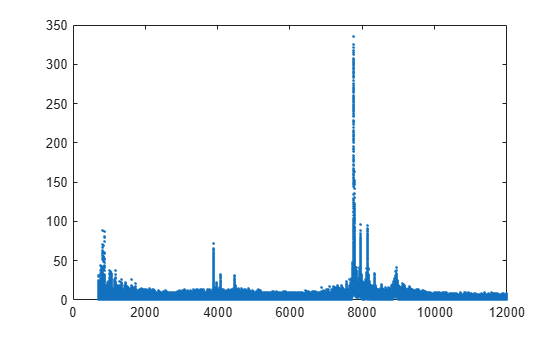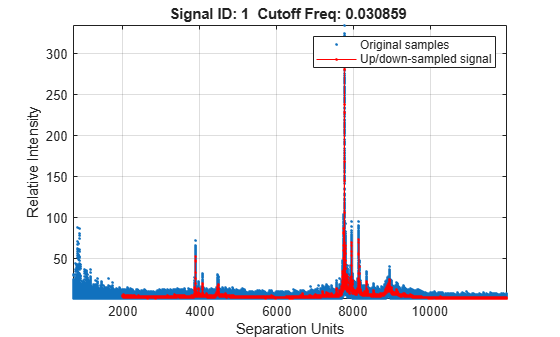# msresample

Resample signal with peaks

## Syntax

```[Xout, Intensitiesout] = msresample(X, Intensities, N) msresample(..., 'Uniform', UniformValue, ...) msresample(..., 'Range', RangeValue, ...) msresample(..., 'RangeWarnOff', RangeWarnOffValue, ...) msresample(..., 'Missing', MissingValue, ...) msresample(..., 'Window', WindowValue, ...) msresample(..., 'Cutoff', CutoffValue, ...) msresample(..., 'ShowPlot', ShowPlotValue, ...) ```

## Arguments

 `X` Vector of separation-unit values for a set of signals with peaks. The number of elements in the vector equals the number of rows in the matrix `Intensities`. The separation unit can quantify wavelength, frequency, distance, time, or m/z depending on the instrument that generates the signal data. `Intensities` Matrix of intensity values for a set of peaks that share the same separation-unit range. Each row corresponds to a separation-unit value, and each column corresponds to either a set of signals with peaks or a retention time. The number of rows equals the number of elements in vector `X`. `N` Positive integer specifying the total number of samples.

## Description

Tip

Use the following syntaxes with data from any separation technique that produces signal data, such as spectroscopy, NMR, electrophoresis, chromatography, or mass spectrometry.

```[Xout, Intensitiesout] = msresample(X, Intensities, N)``` resamples raw noisy signal data, `Intensities`. The output signal has `N` samples with a spacing that increases linearly within the range ```[min(X) max(X)]```. `X` can be a linear or a quadratic function of its index. When you set input arguments such that down-sampling takes place, `msresample` applies a lowpass filter before resampling to minimize aliasing.

For the antialias filter, `msresample` uses a linear-phase FIR filter with a least-squares error minimization. The cutoff frequency is set by the largest down-sampling ratio when comparing the same regions in the `X` and `Xout` vectors.

Tip

`msresample` is particularly useful when you have signals with different separation-unit vectors and you want to match the scales.

```msresample(..., 'PropertyName', PropertyValue, ...)``` calls `msresample` with optional properties that use property name/property value pairs. You can specify one or more properties in any order. Each `PropertyName` must be enclosed in single quotes and is case insensitive. These property name/property value pairs are as follows:

``` msresample(..., 'Uniform', UniformValue, ...)```, when `UniformValue` is `true`, it forces the vector `X` to be uniformly spaced. The default value is `false`.

```msresample(..., 'Range', RangeValue, ...)``` specifies a `1`-by-`2` vector with the separation-unit range for the output signal, `Intensitiesout`. `RangeValue` must be within `[min(X) max(X)`]. Default value is the full range ```[min(X) max(X)]```. When `RangeValue` values exceed the values in `X`, `msresample` extrapolates the signal with zeros and returns a warning message.

```msresample(..., 'RangeWarnOff', RangeWarnOffValue, ...)``` controls the return of a warning message when `RangeValue` values exceed the values in `X`. `RangeWarnOffValue` can be `true` or `false` (default).

```msresample(..., 'Missing', MissingValue, ...)```, when `MissingValue` is `true`, analyzes the input vector, `X`, for dropped samples. The default value is `false`. If the down-sample factor is large, checking for dropped samples might not be worth the extra computing time. Dropped samples can only be recovered if the original separation-unit values follow a linear or a quadratic function of the `X` vector index.

```msresample(..., 'Window', WindowValue, ...)``` specifies the window used when calculating parameters for the lowpass filter. Enter `'Flattop'`, `'Blackman'`, `'Hamming`', or `'Hanning'`. The default value is `'Flattop'`.

```msresample(..., 'Cutoff', CutoffValue, ...)``` specifies the cutoff frequency. Enter a scalar value from `0` to `1` (Nyquist frequency or half the sampling frequency). By default, `msresample` estimates the cutoff value by inspecting the separation-unit vectors, `X` and `XOut`. However, the cutoff frequency might be underestimated if `X` has anomalies.

```msresample(..., 'ShowPlot', ShowPlotValue, ...)``` plots the original and the resampled signal. When `msresample` is called without output arguments, the signals are plotted unless `ShowPlotValue` is `false`. When `ShowPlotValue` is `true`, only the first signal in `Intensities` is plotted. `ShowPlotValue` can also contain an index to one of the signals in `Intensities`.

Tip

LC/MS data analysis requires extended amounts of memory from the operating system.

## Examples

collapse all

This example shows how to resample mass spec data.

Load a MAT-file, included with Bioinformatics Toolbox™, that contains mass spectrometry data, and then extract m/z and intensity value vectors.

```load sample_hi_res; mz = MZ_hi_res; y = Y_hi_res;```

Plot the original data.

`plot(mz, y, '.')`Resample the spectrogram to have 10000 samples between 2000 and maximum m/z value in the data set, and show both the resampled and original data.

`[mz1,y1] = msresample(mz, y, 10000, 'range',[2000 max(mz)],'SHOWPLOT',true);`Introduced before R2006a

## SupportGet trial now